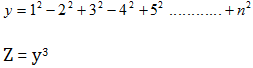# getting started with C++

Hello friends, as mentioned previously here that this time I will be writing about C++ programming, I am going to start it from this post and now on. In this post I will start with the history of C++ language and later on I will let you know how to get started with C++ programming.

# Snake and Ladder game In java

আমরা ছোট বেলায় অনেকেই সাপ লুডু খেলেছি এবং এখনো খেলতে পছন্দ করি। যুগ বদলেছে আজ আমরা মাঠে খেলার বদলে খেলি কম্পিউটার এর ছোট্টখানি পর্দায়। তাই আমিও চেস্টা করেছি আমাদের ছোট্ট বেলার সেই খেলাটিকে ডিজিটাল পর্দায় নিয়ে আসতে। যদিও আমার এই খেলাটি অত্যন্ত সাধারণ মানের এবং এর কোন গ্রাফিক্যাল অস্তিত্ব নেই তবুও এটা কিন্তু ডিজিটাল।

# Some Java program part 6

1. Write a Java program that would input three numbers from the user and print sum, then the first number, then the 2nd number followed by 3rd number. If user enters 10, 20, 30. then output should be 60, 10, 20, 30.
```import java.util.Scanner;
public class Lab8q4{
public static void main ( String [] args ){
int [] number = new int ;
int c = 0, sum=0;
Scanner input = new Scanner ( System.in );
System.out.println(&quot;Enter 3 numbers&quot;);
while(c&lt;3){
number  = input.nextInt();
sum = sum + number ;
++c;
}
System.out.println(&quot;SUM : &quot;+sum);
c=0;
while(c&lt;3){
System.out.println(number);
++c;
}
}
}
```
1. Write a Java program that would input ten numbers from the user and print the ten numbers in reverse order. If user enters 20, 10, 30, 15. Then output should be 15, 30, 10, 20.
```import java.util.Scanner;
public class Lab8q5{
public static void main ( String [] args ){
int [] number = new int ;
int c = 0, sum=0;
Scanner input = new Scanner ( System.in );
System.out.println(&quot;Enter 3 numbers&quot;);
while(c&lt;3){
number  = input.nextInt();
sum = sum + number ;
++c;
}
c=2;
while(c&gt;=0){
System.out.println(number);
--c;
}
}
}
```

# Some Java programs part-5

21. Write a program that will calculate the value of Z  if the expression of y is as follows (n is the input):# Some Java programs part 4

16. Write a program that will read 10 numbers from the user, and then print the first number, the sum of the first 2 numbers, first 3 numbers, and so on up to the sum of 20 numbers.

```/*Time : 5:00pm
* Author: Anik das*/
import java.util.Scanner;
public class Faraz16{
public static void main ( String [] args ){
int c=1, sum=0, number;
Scanner input = new Scanner ( System.in );
while (c&lt;=10){
System.out.println(&quot;Enter Number #&quot;+c);
number = input.nextInt();
sum=sum+number;
System.out.println(&quot;Sum Of 1st &quot;+c+&quot; Number(s) is : &quot;+sum);
c++;
}
}
}
```

# A little about array in Java

I have seen many of my friends to have trouble with the problems in array. So, this time I have selected to write with array…don’t know how much effective it would be to you guys…

First of all, what is array? Normally we can say that array is a static memory allocation. For example we can think an array as a number of conjoined boxes where we can store our desired values of same data type. Now the question may arise that we can use normal variables to store data, why use array? The answer is we should use array because it saves our time and minimizes the memory usage of the machine. For example: suppose that you have to store marks of 5000 students of your university. Now if you want to store those marks using normal variables firstly you have to select 5000 variables (which would be horrible!). Then, you will need to declare each variable (int a, b, c…up to 5000 variables). Than you have to initialize each variable too, haven’t you? In this case if you use array your task will become easier. All you have to do is, firstly you have to declare an array. Secondly, you have to initialize that the array will be consist of 5000 indexes it means the array will consist of 5000 conjoined boxes where the marks will be stored.